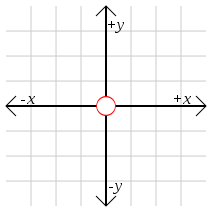# Origin (mathematics)In mathematics, the origin of a Euclidean space is a special point, usually denoted by the letter O, used as a fixed point of reference for the geometry of the surrounding space.

In physical problems, the choice of origin is often arbitrary, meaning any choice of origin will ultimately give the same answer. This allows one to pick an origin point that makes the mathematics as simple as possible, often by taking advantage of some kind of geometric symmetry.

## Cartesian coordinates

In a Cartesian coordinate system, the origin is the point where the axes of the system intersect. The origin divides each of these axes into two halves, a positive and a negative semiaxis. Points can then be located with reference to the origin by giving their numerical coordinates—that is, the positions of their projections along each axis, either in the positive or negative direction. The coordinates of the origin are always all zero, for example (0,0) in two dimensions and (0,0,0) in three.

## Other coordinate systems

In a polar coordinate system, the origin may also be called the pole. It does not itself have well-defined polar coordinates, because the polar coordinates of a point include the angle made by the positive x-axis and the ray from the origin to the point, and this ray is not well-defined for the origin itself.

In Euclidean geometry, the origin may be chosen freely as any convenient point of reference.

The origin of the complex plane can be referred as the point where real axis and imaginary axis intersect each other. In other words, it is the complex number zero.